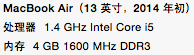# 使用Python进行并发编程

posted @ 2015年3月20日 05:46 in 软件开发 with tags python Concurrent , 1138 阅读

### 并发方式

```import thread
def worker():
print 'Worker'

```import threading
def worker():
print 'Worker'
t.start()```

或者Java Style

```import threading
def __init__(self):
pass
def run():
print 'Worker'

t = worker()
t.start()```

#### 进程 （Process）

```from multiprocessing import Process

def worker():
print 'Worker'
p = Process(target=worker)
p.start()
p.join()```

Python的mutliprocess模块提供了封装好的管道和队列，可以方便的在进程间传递消息。

Python进程间的同步使用锁，这一点喝线程是一样的。

#### 远程分布式主机 （Distributed Node）

• TCP／IP

TCP／IP是所有远程通信的基础，然而API比较低级别，使用起来比较繁琐，所以一般不会考虑

• 远程方法调用 Remote Function Call

RPC是早期的远程进程间通信的手段。Python下有一个开源的实现RPyC

• 远程对象 Remote Object

远程对象是更高级别的封装，程序可以想操作本地对象一样去操作一个远程对象在本地的代理。远程对象最广为使用的规范CORBA，CORBA最大的好处是可以在不同语言和平台中进行通信。当让不用的语言和平台还有一些各自的远程对象实现，例如Java的RMI，MS的DCOM

Python的开源实现，有许多对远程对象的支持

• 消息队列 Message Queue

比起RPC或者远程对象，消息是一种更为灵活的通信手段，常见的支持Python接口的消息机制有

Python下有许多开源的框架来支持分布式的并发，提供有效的管理手段包括：

• Celery是一个非常成熟的Python分布式框架，可以在分布式的系统中，异步的执行任务，并提供有效的管理和调度功能。参考这里

• SCOOP Scalable COncurrent Operations in Python）提供简单易用的分布式调用接口，使用Future接口来进行并发。

• 相比起Celery和SCOOP，Dispy提供更为轻量级的分布式并行服务

• PP （Parallel Python）是另外一个轻量级的Python并行服务， 参考这里

• Asyncoro是另一个利用Generator实现分布式并发的Python框架，

• greenlet提供轻量级的coroutines来支持进程内的并发。

```from greenlet import greenlet

def test1():
print 12
gr2.switch()
print 34

def test2():
print 56
gr1.switch()
print 78

gr1 = greenlet(test1)
gr2 = greenlet(test2)
gr1.switch()```

运行以上程序得到如下结果：

```12
56
34```

eventlet，gevent和concurence都是基于greenlet提供并发的。

eventlet是一个提供网络调用并发的Python库，使用者可以以非阻塞的方式累调用阻塞的IO操作。

```import eventlet
from eventlet.green import urllib2

urls = ['http://www.google.com', 'http://www.example.com', 'http://www.python.org']

def fetch(url):

pool = eventlet.GreenPool()

for body in pool.imap(fetch, urls):
print("got body", len(body))```

```('got body', 17629)
('got body', 1270)
('got body', 46949)```

eventlet为了支持generator的操作对urllib2做了修改，接口和urllib2是一致的。这里的GreenPool和Python的Pool接口一致。

gevent和eventlet类似，关于它们的差异大家可以参考这篇文章

```import gevent
from gevent import socket
urls = ['www.google.com', 'www.example.com', 'www.python.org']
jobs = [gevent.spawn(socket.gethostbyname, url) for url in urls]
gevent.joinall(jobs, timeout=2)

print [job.value for job in jobs]```

`['206.169.145.226', '93.184.216.34', '23.235.39.223']`
• concurence https://github.com/concurrence/concurrence

concurence是另外一个利用greenlet提供网络并发的开源库，我没有用过，大家可以自己尝试一下。

### 实战运用

#### 计算密集型```from math import hypot
from random import random

def test(tries):
return sum(hypot(random(), random()) < 1 for _ in range(tries))```

• 非并发

我们先在单线程，但进程运行，看看性能如何

```from math import hypot
from random import random
import eventlet
import time

def test(tries):
return sum(hypot(random(), random()) < 1 for _ in range(tries))

def calcPi(nbFutures, tries):
ts = time.time()
result = map(test, [tries] * nbFutures)

ret = 4. * sum(result) / float(nbFutures * tries)
span = time.time() - ts
print "time spend ", span
return ret

print calcPi(3000,4000)```

为了使用线程池，我们用multiprocessing的dummy包，它是对多线程的一个封装。注意这里代码虽然一个字的没有提到线程，但它千真万确是多线程。

通过测试我们开（jing）心（ya）的发现，果然不出所料，当线程池为1是，它的运行结果和没有并发时一样，当我们把线程池数字设置为5时，耗时几乎是没有并发的2倍，我的测试数据从5秒到9秒。所以对于计算密集型的任务，还是放弃多线程吧。

```from multiprocessing.dummy import Pool

from math import hypot
from random import random
import time

def test(tries):
return sum(hypot(random(), random()) < 1 for _ in range(tries))

def calcPi(nbFutures, tries):
ts = time.time()
p = Pool(1)
result = p.map(test, [tries] * nbFutures)
ret = 4. * sum(result) / float(nbFutures * tries)
span = time.time() - ts
print "time spend ", span
return ret

if __name__ == '__main__':
p = Pool()
print("pi = {}".format(calcPi(3000, 4000)))```
• 多进程 multiprocess

理论上对于计算密集型的任务，使用多进程并发比较合适，在以下的例子中，进程池的规模设置为5，修改进程池的大小可以看到对结果的影响，当进程池设置为1时，和多线程的结果所需的时间类似，因为这时候并不存在并发；当设置为2时，响应时间有了明显的改进，是之前没有并发的一半；然而继续扩大进程池对性能影响并不大，甚至有所下降，也许我的Apple Air的CPU只有两个核？当心，如果你设置一个非常打的进程池，你会遇到 Resource temporarily unavailable的错误，系统并不能支持创建太多的进程，毕竟资源是有限的。

```from multiprocessing import Pool

from math import hypot
from random import random
import time

def test(tries):
return sum(hypot(random(), random()) < 1 for _ in range(tries))

def calcPi(nbFutures, tries):
ts = time.time()
p = Pool(5)
result = p.map(test, [tries] * nbFutures)
ret = 4. * sum(result) / float(nbFutures * tries)
span = time.time() - ts
print "time spend ", span
return ret

if __name__ == '__main__':
p = Pool()
print("pi = {}".format(calcPi(3000, 4000)))```
• gevent （伪线程）

不论是gevent还是eventlet，因为不存在实际的并发，响应时间和没有并发区别不大，这个和测试结果一致。

```import gevent
from math import hypot
from random import random
import time

def test(tries):
return sum(hypot(random(), random()) < 1 for _ in range(tries))

def calcPi(nbFutures, tries):
ts = time.time()
jobs = [gevent.spawn(test, t) for t in [tries] * nbFutures]
gevent.joinall(jobs, timeout=2)
ret = 4. * sum([job.value for job in jobs]) / float(nbFutures * tries)
span = time.time() - ts
print "time spend ", span
return ret

print calcPi(3000,4000)```
• eventlet （伪线程）

```from math import hypot
from random import random
import eventlet
import time

def test(tries):
return sum(hypot(random(), random()) < 1 for _ in range(tries))

def calcPi(nbFutures, tries):
ts = time.time()
pool = eventlet.GreenPool()
result = pool.imap(test, [tries] * nbFutures)

ret = 4. * sum(result) / float(nbFutures * tries)
span = time.time() - ts
print "time spend ", span
return ret

print calcPi(3000,4000)```
• SCOOP

SCOOP中的Future接口符合PEP-3148的定义，也就是在Python3中提供的Future接口。

```from math import hypot
from random import random
from scoop import futures

import time

def test(tries):
return sum(hypot(random(), random()) < 1 for _ in range(tries))

def calcPi(nbFutures, tries):
ts = time.time()
expr = futures.map(test, [tries] * nbFutures)
ret = 4. * sum(expr) / float(nbFutures * tries)
span = time.time() - ts
print "time spend ", span
return ret

if __name__ == "__main__":
print("pi = {}".format(calcPi(3000, 4000)))```
• Celery

```from celery import Celery

from math import hypot
from random import random

app = Celery('tasks', backend='amqp', broker='amqp://guest@localhost//')
app.conf.CELERY_RESULT_BACKEND = 'db+sqlite:///results.sqlite'

def test(tries):
return sum(hypot(random(), random()) < 1 for _ in range(tries))```

```from celery import group
from tasks import test

import time

def calcPi(nbFutures, tries):
ts = time.time()
result = group(test.s(tries) for i in xrange(nbFutures))().get()

ret = 4. * sum(result) / float(nbFutures * tries)
span = time.time() - ts
print "time spend ", span
return ret

print calcPi(3000, 4000)```

• asyncoro

Asyncoro的测试结果和非并发保持一致。

```import asyncoro

from math import hypot
from random import random
import time

def test(tries):
yield sum(hypot(random(), random()) < 1 for _ in range(tries))

def calcPi(nbFutures, tries):
ts = time.time()
coros = [ asyncoro.Coro(test,t) for t in [tries] * nbFutures]
ret = 4. * sum([job.value() for job in coros]) / float(nbFutures * tries)
span = time.time() - ts
print "time spend ", span
return ret

print calcPi(3000,4000)```

#### IO密集型

IO密集型的任务是另一种常见的用例，例如网络WEB服务器就是一个例子，每秒钟能处理多少个请求时WEB服务器的重要指标。

```from math import hypot
import time
import urllib2

urls = ['http://www.google.com', 'http://www.example.com', 'http://www.python.org']

def test(url):

def testIO(nbFutures):
ts = time.time()
map(test, urls * nbFutures)

span = time.time() - ts
print "time spend ", span

testIO(10)```

Python提供了不同的并发方式，对应于不同的场景，我们需要选择不同的方式进行并发。选择合适的方式，不但要对该方法的原理有所了解，还应该做一些测试和试验，数据才是你做选择的最好参考。Assam Board 4th Clas 说:
2022年9月06日 22:01

Assam Board Model Paper 2023 Class 4 Pdf Download with Answers for English Medium, Bangali Medium, Hindi Medium & Assamese Medium Students for Small Answers, Long Answer, Very Long Answer Questions, and Essay Type Questions to Term1 & Term2 Exams at official website. Assam Board 4th Class Model Paper New Exam Scheme or Question Pattern for Sammittive Assignment Exams (SA1 & SA2): Very Long Answer (VLA), Long Answer (LA), Small Answer (SA), Very Small Answer (VSA), Single Answer, Multiple Choice and etc.(输入验证码)
or Ctrl+Enter